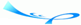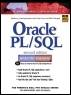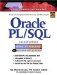# Chapter 6 Conditional Control: CASE Statements

 Team-FlyOracle® PL/SQL® Interactive Workbook, Second EditionBy Benjamin Rosenzweig, Elena Silvestrova Table of Contents Appendix D.  Answers to Test Your Thinking Sections

 1) Create the following script. Modify the script created in this section in Chapter 5 (Question 1 of the Test Your Thinking section). You can use either the CASE statement or the searched CASE statement. Your output should look similar to the output produced by the example created in Chapter 5. A1: Answer: Consider the script created in the section in Chapter 5:` SET SERVEROUTPUT ON DECLARE v_day VARCHAR2(15); v_time VARCHAR(8); BEGIN v_day := TO_CHAR(SYSDATE, 'fmDAY'); v_time := TO_CHAR(SYSDATE, 'HH24:MI'); IF v_day IN ('SATURDAY', 'SUNDAY') THEN DBMS_OUTPUT.PUT_LINE (v_day||', '||v_time); IF v_time BETWEEN '12:01' AND '24:00' THEN DBMS_OUTPUT.PUT_LINE ('It''s afternoon'); ELSE DBMS_OUTPUT.PUT_LINE ('It''s morning'); END IF; END IF; -- control resumes here DBMS_OUTPUT.PUT_LINE ('Done…'); END; `Next, consider the modified version of the script with nested CASE statements. For illustrative purposes, this script uses both CASE and searched CASE statements. All changes are shown in bold letters.` SET SERVEROUTPUT ON DECLARE v_day VARCHAR2(15); v_time VARCHAR(8); BEGIN v_day := TO_CHAR(SYSDATE, 'fmDay'); v_time := TO_CHAR(SYSDATE, 'HH24:MI'); -- CASE statement CASE SUBSTR(v_day, 1, 1) WHEN 'S' THEN DBMS_OUTPUT.PUT_LINE (v_day||', '||v_time); -- searched CASE statement CASE WHEN v_time BETWEEN '12:01' AND '24:00' THEN DBMS_OUTPUT.PUT_LINE ('It''s afternoon'); ELSE DBMS_OUTPUT.PUT_LINE ('It''s morning'); END CASE; END CASE; -- control resumes here DBMS_OUTPUT.PUT_LINE('Done…'); END; `In this exercise, you substitute nested IF statements with nested CASE statements. Consider the outer CASE statement. It uses a selector expression ` SUBSTR(v_day, 1, 1) `to check if a current day falls on the weekend. Notice that it derives only the first letter of the day. This is a good solution when using a CASE statement because only Saturday and Sunday start with letter 'S'. Furthermore, without using the SUBSTR function, you would need to use a searched CASE statement. You will recall that the value of the WHEN expression is compared to the value of the selector. As a result, the WHEN expression must return a similar datatype. In this example, the selector the expression returns a string datatype, so the WHEN expression must also return a string datatype.Next, you use a searched CASE to validate the time of the day. You will recall that, similar to the IF statement, the WHEN conditions of the searched CASE statement yield Boolean values.When run, this exercise produces the following output:` Saturday, 19:49 It's afternoon Done… PLSQL procedure successfully completed. ` 2) Create the following script. Modify the script created in this section in Chapter 5 (Question 2 of the Test Your Thinking section). You can use either the CASE statement or the searched CASE statement. Your output should look similar to the output produced by the example created in Chapter 5. A2: Answer: Consider the script created in the section in Chapter 5:` SET SERVEROUTPUT ON DECLARE v_instructor_id NUMBER := &sv_instructor_id; v_total NUMBER; BEGIN SELECT COUNT(*) INTO v_total FROM section WHERE instructor_id = v_instructor_id; -- check if instructor teaches 3 or more sections IF v_total >= 3 THEN DBMS_OUTPUT.PUT_LINE ('This instructor needs '|| a vacation'); ELSE DBMS_OUTPUT.PUT_LINE ('This instructor teaches '|| v_total||' sections'); END IF; -- control resumes here DBMS_OUTPUT.PUT_LINE ('Done…'); END; `Next, consider modified version of the script with the searched CASE statement instead of the IF-THEN-ELSE statement. All changes are shown in bold letters.` SET SERVEROUTPUT ON DECLARE v_instructor_id NUMBER := &sv_instructor_id; v_total NUMBER; BEGIN SELECT COUNT(*) INTO v_total FROM section WHERE instructor_id = v_instructor_id; -- check if instructor teaches 3 or more sections CASE WHEN v_total >= 3 THEN DBMS_OUTPUT.PUT_LINE ('This instructor needs '|| a vacation'); ELSE DBMS_OUTPUT.PUT_LINE ('This instructor teaches '|| v_total||' sections'); END CASE; -- control resumes here DBMS_OUTPUT.PUT_LINE ('Done…'); END; `Assume that value 109 was provided at runtime. Then the script produces the following output:` Enter value for sv_instructor_id: 109 old 2: v_instructor_id NUMBER := &sv_instructor_id; new 2: v_instructor_id NUMBER := 109; This instructor teaches 1 sections Done… PLSQL procedure successfully completed. `In order to use the CASE statement, the searched CASE statement could be modified as follows:` CASE SIGN(v_total 3) WHEN -1 THEN DBMS_OUTPUT.PUT_LINE ('This instructor teaches '|| v_total||' sections'); ELSE DBMS_OUTPUT.PUT_LINE ('This instructor needs '|| a vacation'); END CASE; `Notice that the SIGN function is used to determine if an instructor teaches three or more sections. You will recall that the SIGN function returns -1 if v_total is less than 3, 0 if v_total equals to 3, and 1 if v_total is greater than 3. In this case, as long as the SIGN function returns -1, the message 'This instructor teaches…' is displayed on the screen. In all other cases, the message 'This instructor needs a vacation' is displayed on the screen. 3) Execute the following two SELECT statements and explain why they produce different output:` SELECT e.student_id, e.section_id, e.final_grade, g.numeric_grade, COALESCE(g.numeric_grade, e.final_grade) grade FROM enrollment e, grade g WHERE e.student_id = g.student_id AND e.section_id = g.section_id AND e.student_id = 102 AND g.grade_type_code = 'FI'; SELECT e.student_id, e.section_id, e.final_grade, g.numeric_grade, NULLIF(g.numeric_grade, e.final_grade) grade FROM enrollment e, grade g WHERE e.student_id = g.student_id AND e.section_id = g.section_id AND e.student_id = 102 AND g.grade_type_code = 'FI'; ` A3: Answer: Consider outputs produced by the following SELECT statements:` STUDENT_ID SECTION_ID FINAL_GRADE NUMERIC_GRADE GRADE ---------- ---------- ----------- ------------- ---------- 102 86 85 85 102 89 92 92 92 STUDENT_ID SECTION_ID FINAL_GRADE NUMERIC_GRADE GRADE ---------- ---------- ----------- ------------- ---------- 102 86 85 85 102 89 92 92 `Consider the output returned by the first SELECT statement. This statement uses the COALESCE function to derive the value of GRADE. It equals the value of the NUMERIC_GRADE in the first row and the value of FINAL_GRADE in the second row.The COALESCE function compares the value of the FINAL_GRADE to NULL. If it is NULL, then the value of the NUMERIC_GRADE is compared to NULL. Because the value of the NUMERIC_GRADE is not NULL, the COALESCE function returns the value of the NUMERIC_GRADE in the first row. In the second row, the COALESCE function returns the value of FINAL_GRADE because it is not NULL.Next, consider the output returned by the second SELECT statement. This statement uses the NULLIF function to derive the value of GRADE. It equals the value of the NUMERIC_GRADE in the first row, and it is NULL in the second row.The NULLIF function compares NUMERIC_GRADE value to the FINAL_GRADE value. If these values are equal, the NULLIF function returns NULL. In the opposite case, it returns the value of the NUMERIC_GRADE.

 Team-FlyTopOracle PL/SQL Interactive Workbook (2nd Edition)
ISBN: 0130473200
EAN: 2147483647
Year: 2002
Pages: 146

Similar book on Amazon

flylib.com © 2008-2017.
If you may any questions please contact us: flylib@qtcs.net